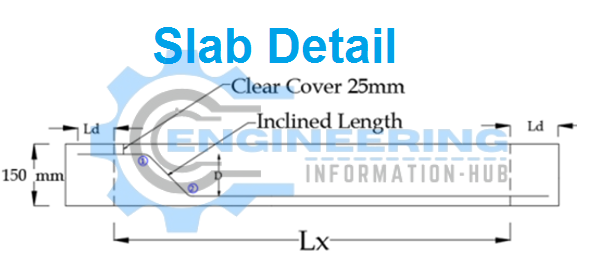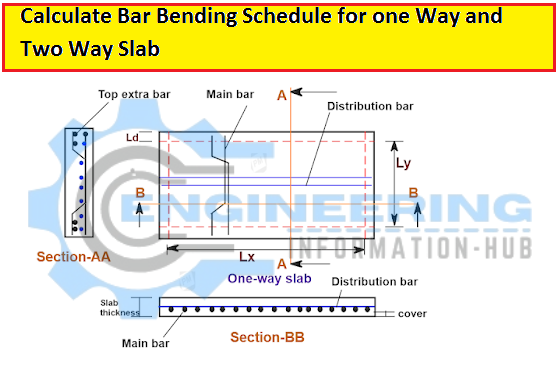Bar Bending Schedule

# Calculate The Bar Bending Schedule For One Way And Two Way Slab

## Calculate The Bar Bending Schedule For One Way And Two Way Slab

Today I have the topic of Calculate The Bar Bending Schedule For One Way And Two Way Slab.| what is the difference between one-way ad two-way slab |Bar Bending Schedule For One Way Slab |Bar Bending Schedule For Two Way Slab|Bar Detail of one Way Slab | Bar Detail of Two Way slab?

### What Is The One Way and Two Way Slab?

Before calculating the bar bending schedule for the slab must be known about the one-way and two-way slabs. one way slab has the main bar or crank bar on the shorter side and a distribution bar on the long side. But the two-way slab has both sides the crank bar which is the difference between one way and a two-way slab.

### Bar Detail on a one-way slabMain bar or crank bar on the shorter side

The distribution bar is on the long side. And this bar is the straight bar

The extra bar has the bottom of the crank bar. And this bar is the straight bar.

### Bar Detail on a two-way slab

The main bar and distribution are the same size same crank bar on both sides.

The extra bar has the bottom of the crank bar on both sides.

### Calculate the Bar Bending Schedule for One Way and Two Way Slab### One way slab

Given data:

Length of slab (along beam) =5000mm=5m

Width of slab (without beam 90degree beam side) =2000mm=2m

Length of extra bar=L/4=2000/4=500

Diameter of main bar/crank bar=16mm

Diameter of top extra bar=16mm

Diameter of distribution bar=12mm

Spacing of main and distribution bar=150mm

Concrete cover bottom and top=20mm

Concrete cover of side=25mm

Solution

### Cutting Length of main bar/crank bar

Cutting Length of main bar/crank bar=clear span+2xLd+crank length -2bends 45degre

Cutting Length of main bar/crank bar=2000+(2x40d)+0.42D-2x45d

Here

Ld=development length

Ld=40d=40×16=640mm

Crank length=0.42D ({D= deapth of slab-2(sides c.c) –dia of bar}

D= 150-2(25)-16=84mm

45 degree bend=1d=16

Now

Cutting Length of main bar/crank bar=2000+(2x40x16)+(0.42×84)-(2×16)

Cutting Length of main bar/crank bar=2000+1280+35.28-32=3283.28mm=3.283m

Number of main bar/crank bar

Number of main bar/crank bar =length of slab/spacing+1

Number of main bar/crank bar =5000/150+1=34.33=35No’s

Total length of main bar/crank ba=length of one bar x number of bar

Total length of main bar/crank bar=3.283×35=114.915m

### Weight of main bar/crank bar

Weight of main bar/crank bar=d²/162.25 x total length

Weight of main bar/crank bar=(16)²/162.25 x 114.915=181.314kg=182kg

### Cutting Length of distribution bar

Cutting Length of distribution bar=length of the slab +2xLd

Ld = 40d=40×12=480mm

Cutting Length of distribution bar=5000+2x40x12=5960mm=5.960m

Number of distribution bar

Number of distribution bar=total width of slab/spacing +1

Number of distribution bar=2000/150+1=14.33=15No’s

Total Length of distribution bar=length of one bar x number of bar

Total Length of distribution bar=5.960×15=89.400m

### Weight of distribution bar

Weight of distribution bar=d²/162.25 x total length

Weight of distribution bar=(12)²/162.25 x 89.400=79.344=80kg

### Top Extra bars

Cutting length of extra bar

Cutting length of extra bar=L/4=2000/4=500

We know

Number of extra bar= Number of the main bar

35=35

The total length of extra bar=length of one extra bar x Number of extra bar

Total length of extra bar=500×35=17500mm=17.500m

### Weight of Extra bar

Weight of Extra bar= d²/162.25 x total length

Weight of Extra bar= (16)²/162.25 x 17.500=27.612=28kg

Total weight of 16mm=182+28=210kg

Total weight of 12mm=80kg

### Calculate The Bar bending Schedule for Two Way slab

Bar bending schedule for two way slab have both sides of the crank bar and is the same procedure that is followed to find the one way slab for the crank bar.

### Load Calculation For Column Beam And Slab Design Calculation

THANKS HAVE A NICE DAY

### Raja Numan

Hi, My name is Engr. Raja Numan author of Engineering Information Hub and I am a Civil Engineer by Profession and I've specialized in the field of Quantity Surveying, Land Surveying as QC Engineer in national and multinational companies of Pakistan & Saudi Arabia.

### One Comment

1.John says:

thx for sharing

Check Also
Close
error: Content is protected !!